# Coordinate Systems

Let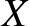,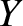,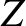be a conventional right-handed Cartesian coordinate system (wheremeasures vertical height).

Let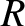,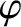,be the corresponding cylindrical coordinate system, where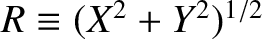and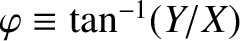. It follows that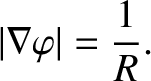(14.1)

Finally, let us define a flux coordinate system: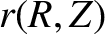,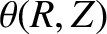,, where [5,14]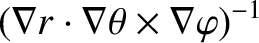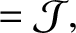(14.2)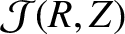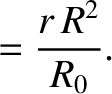(14.3)

(Note that the,,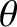,are the same as the corresponding quantities defined in Section 2.10.) Here,is a convenient scale major radius, the magnetic flux-surface label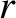has units of length, andis is an angular coordinate that increases by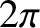radians for every poloidal circuit of the magnetic axis. Let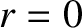correspond to the magnetic axis of the plasma, and let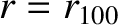correspond to the last closed magnetic flux-surface. Suppose that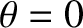on the outboard midplane of the plasma. It follows that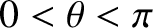below the midplane.